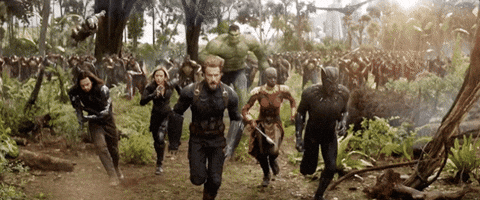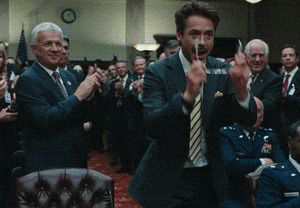Team XenoX

# How to make Dynamic Text Overlays on Images

If you've ever made a webapp, you must be familiar with this scenario. So many times, you find yourself in a situation where you have to put some text on an image. And if the image is uploaded by a user, then it is hard to predict what color of text would go with the image. Consider the following example :The text Rachel Rose looks perfect on a dark background, but what if the background was white? That may cause a problem because you can never predict what a user will upload.

An easy solution is to use a blur filter on the image like this...

``````    .image-blur{
filter: blur(1px);
}
``````

...or make a dark overlay on the image. There many ways you can do, and they all rely on making changes to the image. But what if instead of changing the image, we could change the text color?

"Well, why not?" is what I told myself when this thought crossed my mind recently. So let's explore how to do that in this post.### How to do it?

I have created a simple page to demonstrate this problem. If you wanna learn as you go, just clone this repo.

## Dynalay Demo

A simple way to make a dynamic text overlays on images. Check here

## Prerequisites

Before running this locally you must have these installed

• Node
• Webpack

## Installing

It's built in node so the process to start this is really easy

1. `npm install`
2. `npm run start:dev`

That's it, you will see it running on localhost:8080

## Contributing

Feel free to contribute to this project and treat it like your own. 😊

## Author

Sarthak Sharma

Once cloned, use this commit to check out the unsolved problem.

``````   git checkout 0817434
npm install
npm run start:dev
``````

So currently, we have a webpage that has two types of backgrounds: dark and light. It also has two buttons to switch between them. This is how it looks like in the beginning.To achieve this, we will use the canvas. The idea is to load the image in the canvas and then we will fetch each and every pixel of the image. The color of each pixel will be then converted into its RGB value. So if you average the three values, you will get the lightness of that pixel (read more here). The values will be between 0 (darkest) and 255 (brightest). So by comparing this for each pixel, we will get if the image is dark or not.

Great, now as we understand the algorithm, let's write some code.First, make a function that will load src from the background into a hidden `img` element.

``````    const isDark = (src)=>{
//create a hidden img element
let img = document.createElement("img");
img.src = src;
img.style.display = "none";
document.body.appendChild(img);
}
``````

Then draw the image on canvas using

``````const isDark = (src)=>{

//create a hidden img element
let img = document.createElement("img");
img.src = src;
img.style.display = "none";
document.body.appendChild(img);

// create canvas
let canvas = document.createElement("canvas");
canvas.width = this.width;
canvas.height = this.height;

let ctx = canvas.getContext("2d");
ctx.drawImage(this,0,0);
}
}
``````

Now we need to get the RGB value of each pixel. For that, let's use `getImageData`

``````    let imageData = ctx.getImageData(0,0,canvas.width,canvas.height);
``````

The output will be something likeGreat! Now we have the data, but it's a huge array in which each pixel's RGB value is separate like thisSo we have to loop through four of them together like

``````        let imageData = ctx.getImageData(0,0,canvas.width,canvas.height);
let data = imageData.data;
let r,g,b,avg;
for(let x = 0, len = data.length; x < len; x+=4) {
r = data[x];
g = data[x+1];
b = data[x+2];
avg = Math.floor((r+g+b)/3);
}
``````

`avg` now has the average RGB value of each pixel. Next, add the average of all the pixels and divide that by total pixels in the image to get the average brightness.

``````       let brightness = Math.floor(colorSum / (this.width*this.height));
``````

So the final function will look something like this:

``````const isDark = (src) => {
return new Promise((resolve, reject) => {
//create a hidden img element
let img = document.createElement("img");
img.src = src;
img.style.display = "none";
document.body.appendChild(img);

let colorSum = 0;
// create canvas
let canvas = document.createElement("canvas");
canvas.width = this.width;
canvas.height = this.height;

let ctx = canvas.getContext("2d");
ctx.drawImage(this, 0, 0);

let imageData = ctx.getImageData(0, 0, canvas.width, canvas.height);
let data = imageData.data;
let r, g, b, avg;
for (let x = 0, len = data.length; x < len; x += 4) {
r = data[x];
g = data[x + 1];
b = data[x + 2];

avg = Math.floor((r + g + b) / 3);
colorSum += avg;
}

let brightness = Math.floor(colorSum / (this.width * this.height));
resolve(brightness >= 128 ? false : true);
}
});
}
``````

Let's use this function to check if the image is dark or not.

``````    isDark('./images/dbg.jpg').then((data) => {
});
``````

But something is missing. Aha, some style!

``````.dynamic-header {
transition: color 2s;
}
``````Now it's perfect.

#### Conclusion

Hope you guys liked this small experiment and learned something useful from it. There are many ways to achieve the same results, so feel free to comment below if you have one. You may or may not want to use this in your real projects, but I am thinking of making an npm package, to take this to the next level. If you wanna join, DM me @sarthology or just comment below.

Okay guys! Will see you next time. Thanks for reading this.### DiscussionAndrew Bone

It's these 3 lines that I don't like.

``````{
background-clip: text;
color: transparent;
filter: invert(1) grayscale(1) contrast(9);
}
``````

What we're doing is

• removing bits of the span not covered by text
• making the text invisible
• applying filters to the span
• inverting the colour
• setting it to grayscale
• setting the contrast high so we just get black and white.

But it doesn't know where the text is over the image, so it gets the colours from the top left corner.Ken Bellows

Nah, that's not hacky, that's brilliant.
Still not hacky.
Still loving it.
Grayscale? Such a cool idea.
And contrast to finish. Perfect. Why is any of this hacky?

"But it doesn't know where the text is over the image, so it gets the colours from the top left corner." -- Ahhhhh.... and that's where the hack hits the fan.

So close!Jon Randy

Far from perfect. The `isDark` function is extremely naive. For example - if the image has a patch of light or dark that lines up with the text, and the rest of the image is of the opposite 'lightness', you are going to end up with unreadable text again.

What you need to do is test the part of the image that is actually underneath the textEsteban Hernández

Would it be possible to identify the subset of pixels that represent the area under the text from the image data? Then we could reapply the algorithm to a smaller size making it significantly less complex. My main concern with the original implementation would be the cost of scanning a high-resolution image. The profiling algorithm could probably be best applied after the upload on the upload service itself and store the profiling data with the image then we can just fetch the image and it's profiling data and don't have to scan it on client's device.Ken Bellows

It's not too bad as long as you know (or can get) three things:

• the size the image will be when rendered
• the pixel position of the text relative to the top-left corner of the image
• the approximate height and width of the text when rendered

That last bit is the hard part, especially if there's any line breaks involved. But after you have those, it's basically this:

``````const start = { x: img left + text rel left, y: img top + text rel top }
const end = { x: img left + text rel left + text width, y: img top + text rel top + text height }
for (const x = start.x; x < end.x; x++) {
for (const y = start.y; y < end.y; y++) {
const p = (canvas.width * y + x) * 4
const [r, g, b] =[].slice.call(data, p, p+3)
// fancy logic of choice
}
}
``````Mihail Malo

I'd suggest a shadow/outline for most practical uses.
For one thing, you are calculating the average of the whole image.
But imagine if most of the image is white, while exactly where the text goes is a black rectangle?
Then you have to calculate that exact position.
But what if that area is striped?

@link2twenty 's "hacky" CSS solutions will probably be more performant AND more effective, being able to invert the image color with the text.Bassem Ibrahim👨‍💻🇪🇬

Totally awesome! I faced this issue quite alot, always fixed it using a darker overlay on the image ( the easy way ). Would totally like to join on the npm package. But i am wondering what would happen if its a high res image. Would it take a while to go through all the pixels ?